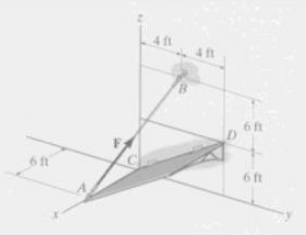# Determine the magnitude of force F in cable AB in order to produce a moment of 500 lb-ft about...

## Question:

Determine the magnitude of force F in cable AB in order to produce a moment of 500 lb-ft about the hinged axis CD.## Moment

When a force is applied to a system, then the product of force and the distance at which the force is applied is termed as a moment. The moment is a measure of force that is able to start the rotary motion when applied to the system.

Become a Study.com member to unlock this answer! Create your account

List down the given data

• The magnitude of the moment of force is {eq}M = 500\;{\rm{lb}} \cdot {\rm{ft}} {/eq}.
• The position vector of point A is...Torque in Physics: Equation, Examples & Problems

from

Chapter 3 / Lesson 13
32K

After watching this video, you will be able to explain what torque is and use an equation to calculate torque in simple situations. A short quiz will follow.